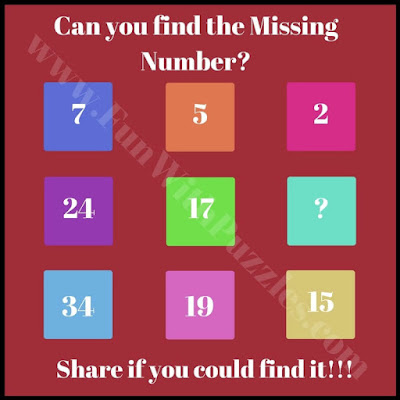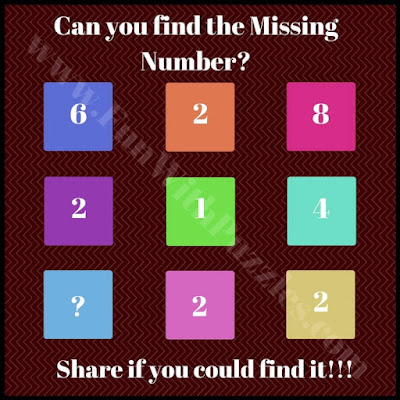There are so many riddles for kids on this website. Some of these riddles are visual puzzles in which one has to use one's observational skills to solve the riddles. Many of these riddles are mathematical which test's one's maths abilities to solve given puzzles. Some are logical riddles that will require some out of box thinking to get the required answer. Adding to these puzzle types is Maths Sequence Puzzles in which one has to find the missing number in the given set of numbers.
In each of these riddles, 9 boxes are given which each containing one number. These numbers are related to each other with some pattern. You have to deduct the hidden pattern and has to find the missing number which will replace the question mark.
Are you ready to find the missing number? Answers to these riddles are given at the end. Do post your answers along with your explanation in the comment section. Also do share it with your friends on Facebook, Twitter, or Whatapps. There are many easy sharing options provided on each page.1. Can you find the missing number?2. Easy maths series question3. Quick math sequence riddle4. Tough maths series riddle5. Tricky maths brain teaser

Answers to these riddles are given below

A1. 7

A2. 15

A3. 8

A4. 11

A5. 3